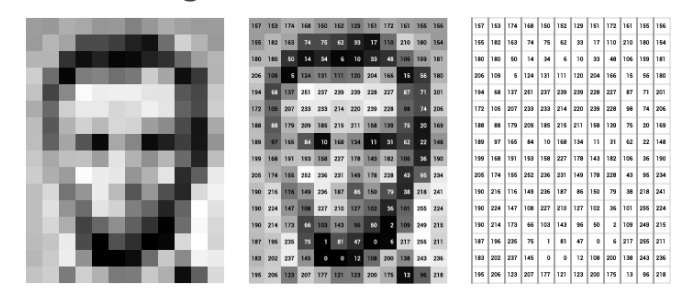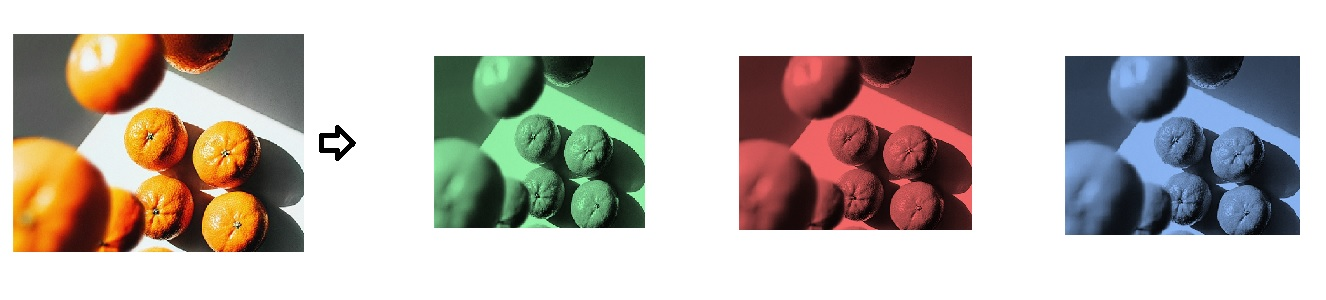# What are the images?

`lang: en_US`

Tip: Participate in Deep Learning Course Certification Quiz to test your knowledge.

## What are images exactly?

Unstructured data, such as text or image data, is commonly employed with deep learning algorithms. And, before working with any form of data, it is necessary to have a thorough understanding of the subject. So, in this session, we'll talk about photos and see how they're saved on a computer. A matrix is a representation of a typical image. Pixel intensity values are represented by the values of this matrix. A higher number indicates a brighter pixel, whereas a lower number indicates a darker pixel. Each color component, such as red, green, and blue, has its channel in a color image. Even though this is the most popular technique to display an image.

## How are black-and-white or grayscale images saved on a computer?

Pixels are little boxes. The expression "the image's dimension is X * Y" is frequently used. What exactly does this imply? This indicates that the image's dimension is simply the number of pixels across the image's height(x) and width(y) dimensions. The below image will show how the image is stored in the format of pixels.Source: https://towarddatascience.com

Even though we see an image above, the computer stores it as a series of numbers. The intensity of the pixels is represented by these pixel values. Pixel values for grayscale or black-and-white images range from 0 to 255.

## On a computer, how do colored graphics become saved?

The image is composed of many colors. Almost all colors can be generated from the three primary colors- Red, Green, and Blue. We can say that each Color image is composed of these three colors or 3 channels- Red, Green, and Blue. In a colored image, this means that there will be three matrices or channels.RGB Image

Each of these metrics will have values ranging from 0 to 255, with each number representing the intensity of the pixels or the red, green, and blue hues. Finally, all of these channels or matrices are stacked so that the image's shape is preserved, and when it is loaded into a computer will be N *M *3, where N is the number of pixels in height, M denotes the number of pixels in width, and 3 denotes the number of channels.

We've seen the following things in this lesson:

• Fundamentals of image formation

We're going to see in the next lesson:

• Digital Video fundamentals.

#### MATLAB Helper ®

Use Website Chat or WhatsApp at +91-8104622179